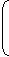# Exam Mission

Exam Information| Govt Jobs.| Exam Preparation | Exam Study Material |Exam Date|Material PDF

1. Let Principal = P, Rate = R% per annum, Time = n years.
2. When interest is compound Annually:
 Amount = P1 + Rn 100
3. When interest is compounded Half-yearly:
 Amount = P1 + (R/2)2n 100
4. When interest is compounded Quarterly:
 Amount = P1 + (R/4)4n 100
5. When interest is compounded Annually but time is in fraction, say 3years.
 Amount = P1 + R3 x1 +R100 100
6. When Rates are different for different years, say R1%, R2%, R3% for 1st, 2nd and 3rd year respectively.
 Then, Amount = P1 + R11 + R21 + R3. 100 100 100
7. Present worth of Rs. x due n years hence is given by:
Present Worth =x.1 + R100